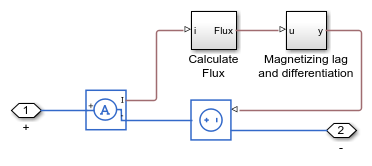# Nonlinear Inductor

This example shows an implementation of a nonlinear inductor where the inductance depends on the current. For best numerical efficiency, the underlying behavior is defined in terms of a current-dependent flux. In order to differentiate the flux to get voltage, a magnetizing lag is included. Simulation results are relatively insensitive to this lag provided that it is at least an order of magnitude faster than the fastest frequency of interest. Parameters for the source and lag are defined in the MATLAB® workspace so that the expected voltage can be calculated analytically.

Here the nonlinear flux-current relationship is defined by a tanh curve. This exhibits the typical curve shape whereby the flux saturates for large currents due to saturation of (for example) an iron core. To model a specific flux-current relationship, replace the tanh function with a PS Lookup Table (1D) from the Simscape™ Foundation Library.

### Model### Nonlinear Inductor Subsystem### Simulation Results from Simscape Logging